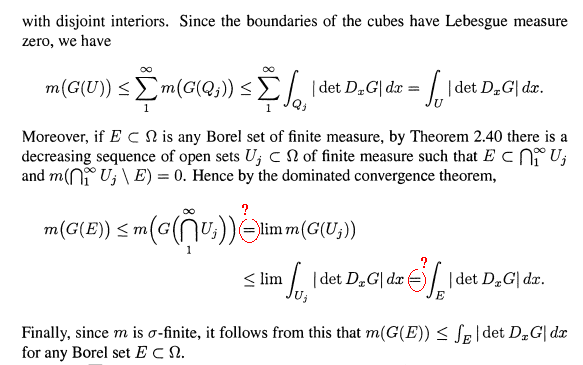# A Question in a proof on Lebesgue integral under diffeomorphism

This question comes from proof of Theorem 2.47 in Folland's "real analysis: modern techniques and their applications", second edition. In particular, the question lies in the inequalities in line 7 and 8 in page 76. The first equality is an application of measure property "continuity from above". But for this property to be applicable, $$m(G(U_1))$$ (or $$m(G(U_j))$$ for at least one of j>1) must be finite. If we can prove that $$\int_{U_1}|\det D_x G|dx$$ is finite, we can derive this result by the inequality in line 3. Secondly, to use dominated convergence theorem to obtain the last "=", $$|\det D_x G|$$ must be integrable on $$U_j$$, that is, $$\int_{U_j}|\det D_x G|dx$$ must be finite. But I can not prove this. I tried to show that $$|\det D_x G|$$ is bounded on $$U_j$$, but $$U_j$$ is only an open set, although it has finite measure. Could you please help me prove that the integral $$\int_{U_j}|\det D_x G|dx$$ is finite? Thanks!
The following image contains part of this proof, for reference.